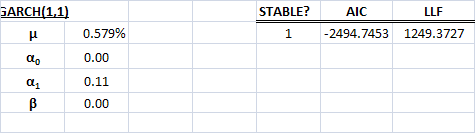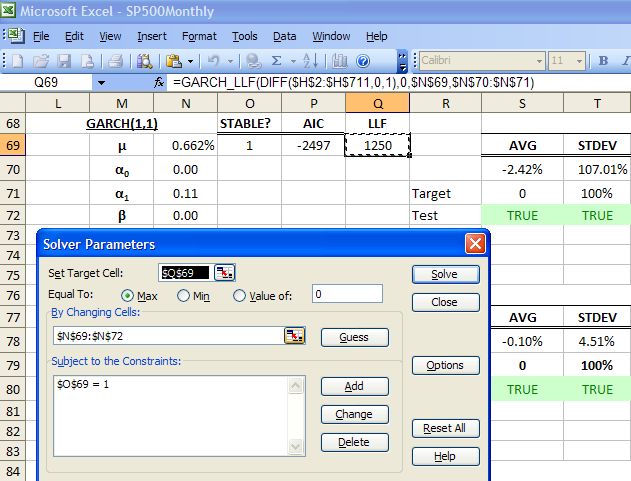# Generating a Model's Coefficients in Excel

## Question:

Is there a way to generate the model's coefficients in Excel?

YES, you can use the Solver add-in that ships with Microsoft Excel. If you don't have enabled, go to the Add-Ins manager and enable it.

1. describe the model you are trying to fit. This is done by guessing the initial coefficients of the model, and compute the log-likelihood (*_LLF()) of the model.2. Designate the cell that holds the log-likelihood as the Target cell, set equal to "Max", cells that hold model coefficients as "Changing-cells", and click on "Solve".3. Using *_CHECK (GARCH_CHECK for GARCH model, ARMA_CHECK for ARMA model, etc.), examine the coefficients whether they yield a stable model. Add a constraint to the solver by designating this cell equals to one(1).4. Now, click on the "Solve" button on the Solver dialog box. The Solver will search for a set of optimal coefficients.
5. Upon completion, the solver will report an optimal solution is found. Click "OK".6. In the rare occasions where a solution can't be found, change your initial guess of the coefficients, and try again.